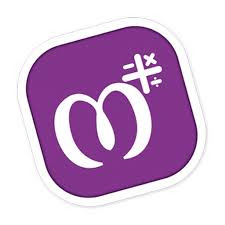## Saturday, 1 February 2020

### Download MAthry A way of Learning Math Supper Application from here

Speed up mathematical calculations with Mathry!

A Simple yet amazing math application for students, teachers and parents.

Increase your brainpower using thousands of mathematical questions/quiz.Excellent for preschool and grade school kids to learn basic arithmetic ideas like Addition, Subtraction, Multiplication, Division, Decimals, Fractions, pure mathematics so on.Learn these basic operations:
Subtraction
Multiplication
Division

Other Features:
Worksheet Generator (Teachers or Parents can use for an exam)
Basic Operations on Number Base
Fractions and Decimals
Mixed Operators
Percentage
Square
Square Root
Cube
Cube Root
Find Missing
and Much More!

Languages available:
English
Spanish
Hindi
Gujarati